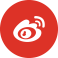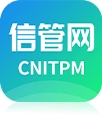﻿ 系统集成项目管理工程师案例分析每日一练试题（2021/5/21） - 每日一练 - 信管网

# 信管网每日一练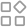导航

## 系统集成项目管理工程师案例分析每日一练试题（2021/5/21）

2021年05月22日来源：信管网 作者:cnitpm［说明］［问题1］（10分）

（1）请计算活动H、G的总浮动时间和自由浮动时间。

（2）请指出该项目的关键路径。

（3）请计算该项目的总工期。

［问题2］（3分）

［问题3］（10分）

（1）请计算该项目的完工预算BAC。

（2）请计算该时点计划值PV、挣值EV、成本绩效指数CPI、进度绩效指数SPI。

［问题4］（3分）

2、会，进度有些落后，按进度网络图，活动e应该要完成100％ 3、bac=27000 pv=2000+3000+2500+3000+2000+2000+2000=16500 ev=2000+3000+1250+3000+1000+2000+2000=14250 ac=2000+4000+2000+2500+1000+2000+2000=15500 cpi=ev/ac=14250/15500=0.92 spi=ev/pv=0.86 4、eac=ac+bac-ev=15500+27000-14250=28250

【问题1】 （1） 活动h的总浮动时间=0天，自由浮动时间=0天 活动g的总浮动时间=3天，自由浮动时间=3天 （2） 该项目的关键路径有两条，分别是 a b c i j a d e h i j （3） 项目工期为：17天 【问题2】 活动c在关键路径上，按照计划，第9天结束c的完成情况为（9-2-4）/6=50％，符合计划 活动e在关键路径上，按照计划，第9天结束应完全完成，现实是只完成了50％,比计划延迟 项目工期会受影响，可能会延迟。 【问题3】 （1）bac=2000+3000+5000+3000+2000+2000+2000+3000+2000+3000=27000元 （2）pv=2000+3000+2500+3000+2000+2000+2000=16500元 ev=15500元 ac=15500元 cpi=ev/ac=1 spi=ev/pv=0.94 【问题4】 按照非典型偏差 eac=ac+bac-ev=27000元

h的自由浮动时间t=0 h的总浮动时间t=0 g的自由浮动时间t=1 g的总浮动时间t=1 关键路径adehij abcij 总工期17天 工期会受到影响，e活动9天结束应该完成，且e在关键路径上。 pv=16500 ev=15500 ac=16000 cpi=ev/ac=96.875％ spi=ev/pv=93.94％ eac=etc+ac=bac-ev=+ac=25000-15500+16000=25500

•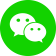•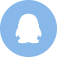•